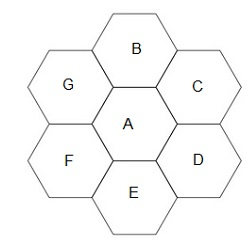## Page

### Hexagonal geometry cell

Having studied the cellular topology and the concept of employing cellular architecture to increases the cellular capacity, serve subscriber demand so we will consider to characteristics the interference in a cellular topology.

A hexagonal cell is one of the closest approximation of a circle to shown in the figure. It is being typically used for the system.

So now let us talk about why we have to choose hexagonal shape summarized below :
• Basically hexagonal is close approximation the circular radiation pattern in an omnidirectional base station antenna.
• Hexagonal allow easy and manageable analysis in a cellular system.
• In a circular pattern, adjacent circles can have gaps in between or can create overlapping regions.
• Hexagonal geometry is used then only less number of a cell can cover the entire market.Hexagonal cell
Having studied the cellular topology and the concept of employing cellular architecture to increases the cellular capacity, serve subscriber demand so we will consider to characteristics the interference in a cellular topology.

A hexagonal cell is one of the closest approximation of a circle to shown in the figure. It is being typically used for the system.

So now let us talk about why we have to choose hexagonal shape summarized below :
• Basically hexagonal is close approximation the circular radiation pattern in an omnidirectional base station antenna.
• Hexagonal allow easy and manageable analysis in a cellular system.
• In a circular pattern, adjacent circles can have gaps in between or can create overlapping regions.
• Hexagonal geometry is used then only less number of a cell can cover the entire market.Hexagonal cell